TitleCollege Algebra
Answer/Discussion to Practice Problems
Tutorial 32: Graphs of Functions, Part II:
Domain/Range, Vertical Line Test, Increasing/Decreasing/Constant Functions, Even/Odd Functions, and Greatest Integer FunctionAnswer/Discussion to 1a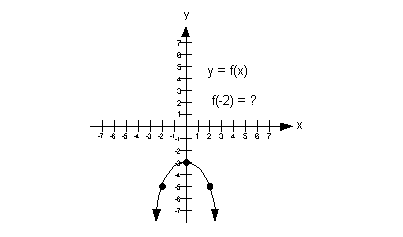a) Domain We need to find the set of all input values.  In terms of ordered pairs, that correlates with the first component of each one.  In terms of this two dimensional graph, that corresponds with the x values  (horizontal axis).  Since that is the case, we need to look to the left and right and see if there are any end points.  In  this case, note how there are arrows on both ends of the graph and no end points.  This means that the graph goes on and on forever in both directions.  This means that the domain is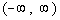.

 b) Range We need to find the set of all output values.  In terms of ordered pairs, that correlates with the second component of each one. In terms of this two dimensional graph, that corresponds with the y values (vertical axis).  Since that is the case, we need to look up and down and see if there are any end points.  In this case, note how the graph has a upper endpoint of y = -3 and it has arrows going down from that. This means that the range is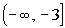.

 c) x-intercept If the x-intercept is where the graph crosses the x-axis, what do you think the x-intercept is for this function? If you said there is none, you are right.  Since the graph never crosses the x-axis, then there is no x-intercept.

 d) y-intercept If the y-intercept is where the graph crosses the y-axis, what do you think the y-intercept is for this function? If you said y = -3 you are correct.  The ordered pair for this y-intercept would be (0, -3).

 e) Functional value indicated If the functional value correlates with the second or y value of an ordered  pair what is f(-2)? If you said f(-2) = -5 , then give yourself a pat on the back.  The functional value at x = -2 is -5.  The ordered pair for this would be (-2, -5).Answer/Discussion to 1b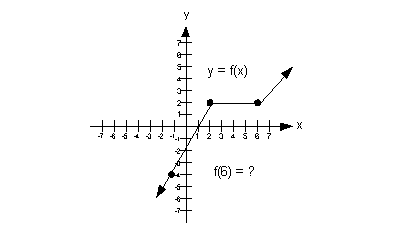a) Domain We need to find the set of all input values.  In terms of ordered pairs, that correlates with the first component of each one.  In terms of this two dimensional graph, that corresponds with the x values  (horizontal axis).  Since that is the case, we need to look to the left and right and see if there are any end points.  In this case, note how there are no endpoints and the graph goes on and on forever in both directions.  This means that the domain is.

 b) Range We need to find the set of all output values.  In terms of ordered pairs, that correlates with the second component of each one. In terms of this two dimensional graph, that corresponds with the y values (vertical axis).  Since that is the case, we need to look up and down and see if there are any end points.  In this case, note how there are no endpoints and the graph goes on and on forever in both directions. This means that the range is.

 c) x-intercept If the x-intercept is where the graph crosses the x-axis, what do you think the x-intercept is for this function? If you said x = 1 you are correct.  The ordered pair for this x-intercept would be (1, 0).

 d) y-intercept If the y-intercept is where the graph crosses the y-axis, what do you think the y-intercept is for this function? If you said y = -2 you are correct.  The ordered pair for this y-intercept would be (0, -2).

 e) Functional value indicated If the functional value correlates with the second or y value of an ordered  pair what is f(6)? If you said f(6) = 2 , then give yourself a pat on the back.  The functional value at x = 6 is 2.  The ordered pair for this would be (6, 2).Answer/Discussion to 2a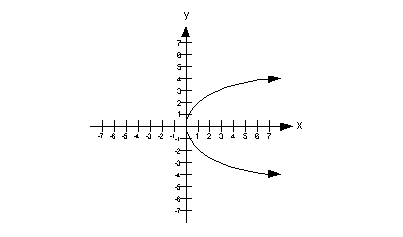This graph would not pass the vertical line test because there is at least one place on it that we could draw a vertical line and intersect it in more than one place.  In fact, there are a lot of vertical lines that we can draw that would intersect it in more than one place, but we only need to show one to say it is not a function. The graph below shows one vertical line drawn through our graph that intersects it in two places: (1, 2) and (1, -2).  This shows that the input value of 1 associates with two output values, which is not acceptable in the function world.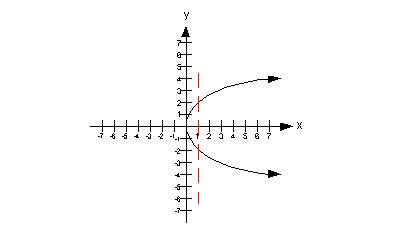Therefore, this is not a graph of a function.Answer/Discussion to 2b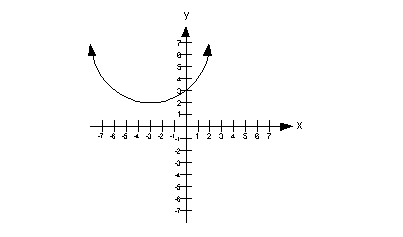This graph would pass the vertical line test, because there would not be any place on it that we could draw a vertical line and it would intersect it in more than one place. Therefore, this is a graph of a function.Answer/Discussion to 3a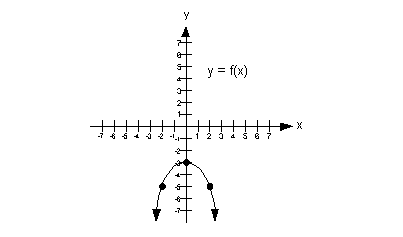a) Increasing A function is increasing in an interval when it is going up left to right in that interval?  With that in mind, what interval, if any, is this function increasing? If you said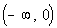, you are correct.  Note how the function is going up left to right, from negative infinity to x = 0.   Below shows the part of the graph that is increasing: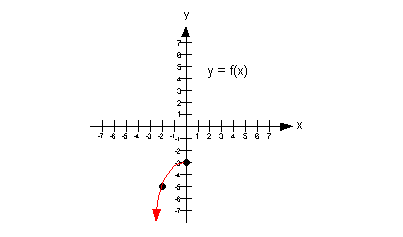b) Decreasing A function is decreasing in an interval when it is going down left to right in that interval?  With that in mind, what interval, if any, is this function decreasing? If you said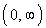, you are right on.  Note how the function is going down left to right starting at x = 0 and everywhere to the right of that.   Below shows the part of the graph that is decreasing: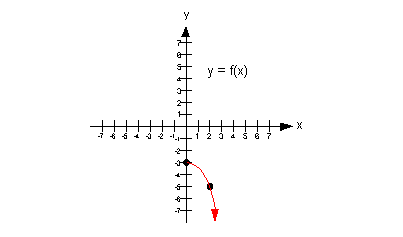c) Constant A function is constant in an interval if it is horizontal in the entire interval.  With that in mind, what interval, if any, is this function constant? If you said it is never constant, pat yourself on the back.  Note how the function is never a horizontal line.Answer/Discussion to 3b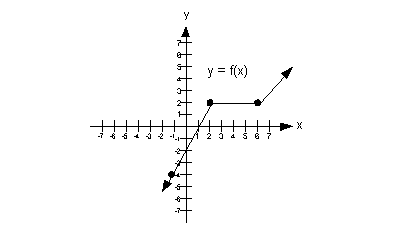a) Increasing A function is increasing in an interval when it is going up left to right in that interval?  With that in mind, what interval, if any, is this function increasing? If you said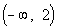or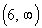, you are correct.  Note how the function is going up left to right, from negative infinity to x = 2 and also starting at x = 6 and everywhere to the right of that.   Below shows the part of the graph that is increasing: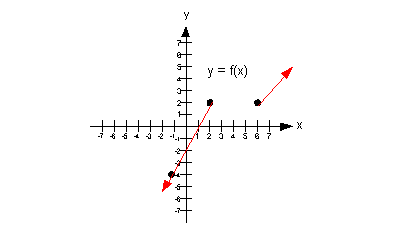b) Decreasing A function is decreasing in an interval when it is going down left to right in that interval?  With that in mind, what interval, if any, is this function decreasing? If you said that it was never decreasing you are right.  The graph never goes down left to right.

 c) Constant A function is constant in an interval if it is horizontal in the entire interval.  With that in mind, what interval, if any, is this function constant? If you said (2, 6), pat yourself on the back.  Note how the function is horizontal starting at x = 2 all the way to x = 6.   Below shows the part of the graph that is constant: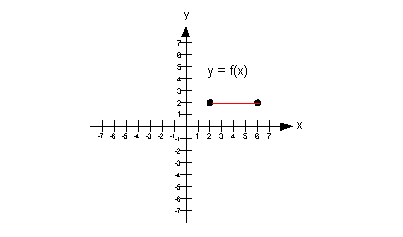Answer/Discussion to 4aNote how this graph is symmetric about the y-axis: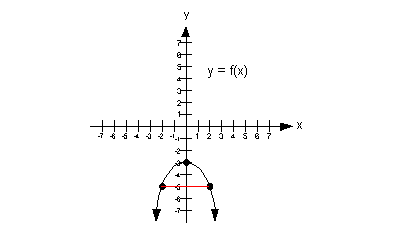Do you know what that means? It means that this function is even.Answer/Discussion to 4bNote how the graph is neither symmetric about the y-axis nor the origin.  Do you know what that means? It means that this function is neither even nor odd.Answer/Discussion to 5a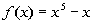To determine if this function is even, odd, or neither,  we need to replace x with -x and compare f(x) with f(-x):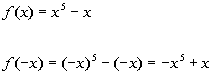Even? A function is even if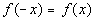for all x in the domain of f.  With that in mind, is this function even? If you said no, you are correct.  Note how both of their terms have opposite signs, so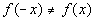.

 Odd? A function is odd if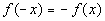for all x in the domain of f.  With that in mind, is this function odd? If you said yes, you are right.  Looking at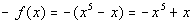, note how all of the terms of f(-x) and -f(x)  match up, so. Final answer: The function is odd.Answer/Discussion to 5b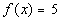To determine if this function is even, odd, or neither,  we need to replace x with -x and compare f(x) with f(-x):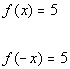Even? A function is even iffor all x in the domain of f.  With that in mind, is this function even? If you said yes, you are correct.  Note how all of the terms of f(x) and f(-x) match up, so. Final answer: The function is even.Answer/Discussion to 5c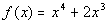To determine if this function is even, odd, or neither,  we need to replace x with -x and compare f(x) with f(-x):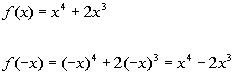Even? A function is even iffor all x in the domain of f.  With that in mind, is this function even? If you said no, you are correct.  Note how their second terms have opposite signs, so.

 Odd? A function is odd iffor all x in the domain of f.  With that in mind, is this function odd? If you said no, you are right.  Looking at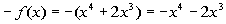, we see that the signs of the first terms of f(-x) and -f(x) don’t match, so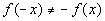.

 Since we said no for both even and odd, that leaves us with our answer to be neither. Final answer: The function is neither even nor odd.Answer/Discussion to 6a f(-9.1)

 We need to ask ourselves, what is the greatest integer that is less than or equal to -9.1? If you said -10, you are correct.   Be careful on this one.  We are working with a negative number.  -9 is not a correct answer because -9 is not less than or equal to -9.1, it is greater than -9.1. Final answer: -10

Last revised on June 18, 2010 by Kim Seward.
All contents copyright (C) 2002 - 2010, WTAMU and Kim Seward. All rights reserved.